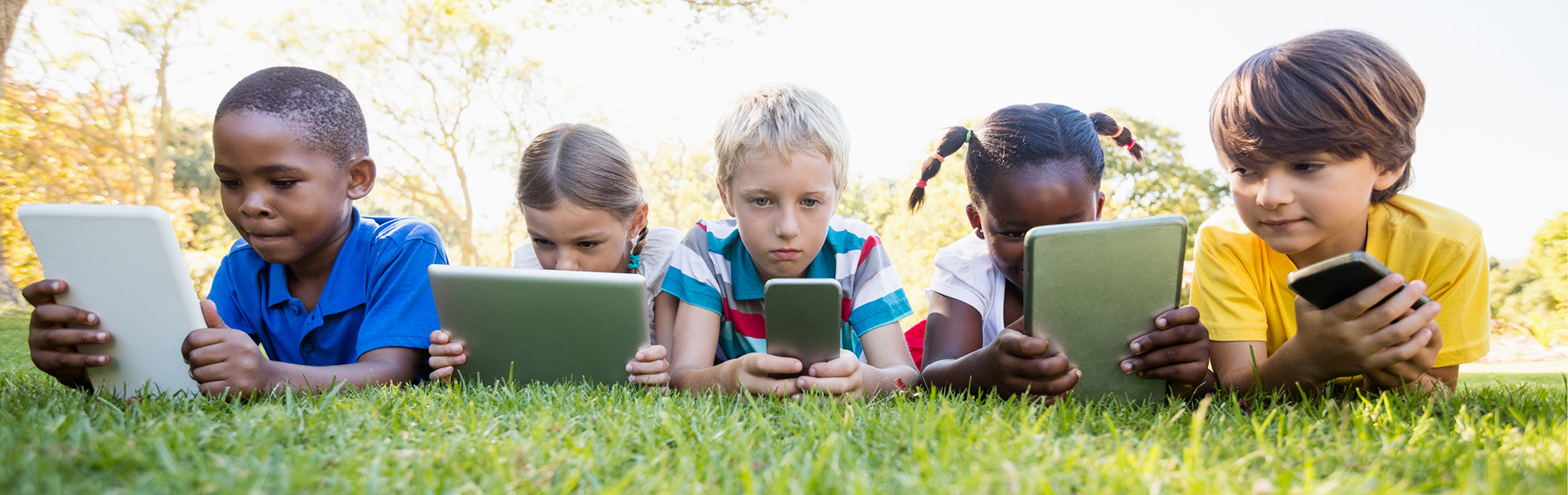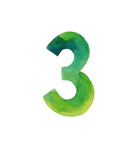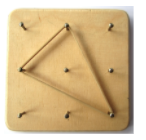# Area and Perimeter with Geoboards

Empowered Learning, Computational Thinking### Overview​

Math Learning Center Geoboard is a manipulative tool students use in geometry to explore the basic concepts of polygons. It also helps with the discovery of area and perimeter. It is a board that contains a certain number of nails in which rubber bands go around to create the shape.

Students will:

• Be able to create various line segments and polygons by stretching the bands around the Geoboard pegs.
• Be able to calculate the perimeter and the area.
• Be able to write equations and expressions with the text tool.

Vocabulary Words:

1. Geometry: Geometry is the study of shapes.

2. Geoboard: A Geoboard is a manipulative tool used in geometry to study the basic shapes.

3. Area: Area is a surface measurement

4. Perimeter: Perimeter is the boundary of a closed geometric figure.

5. Polygon: A polygon is a plane figure with at least three straight sides and angles.

To prepare for this lesson:

1. Students should be familiar with geometry terms.

2. The teacher should introduce the students to the Math Learning Center and the tools to use the Geoboard. View the videos below for assistance.

See Accommodations Page and Charts on the 21things4students.net site in the Teacher Resources.

Directions for this activity:

1. Have students log onto the Math Learning Center.

2. Open Web App (or application appropriate for device).

3. Use rubber bands to create polygons.

4. Use tools to calculate the area and perimeter.
• Drawing tool to label the sides.
• Calculator to calculate the area if needed.

Different options for assessing the students:

• Observations
• Check for understanding
• Assess students with prepared polygons to find the area and perimeter.
• Students demonstrate their model on the projector for the class.
• Math talks discussing how to calculate area and perimeter.
• Give the students a given shape and have them calculate the area and/or perimeter, name of a polygon on an exit ticket.

MITECS: Michigan adopted the "ISTE Standards for Students" called MITECS (Michigan Integrated Technology Competencies for Students) in 2018.

Empowered Learning
1a. Students articulate and set personal learning goals, develop strategies leveraging technology to achieve them and reflect on the learning process itself to improve learning outcomes.
1b. Students build networks and customize their learning environments in ways that support the learning process.
1c. Students use technology to seek feedback that informs and improves their practice and to demonstrate their learning in a variety of ways.
1d. Students understand the fundamental concepts of technology operations, demonstrate the ability to choose, use and troubleshoot current technologies and are able to transfer their knowledge

Computational Thinking
5a. Students formulate problem definitions suited for technology assisted methods such as data analysis, abstract models and algorithmic thinking in exploring and finding solutions.
5c. Students break problems into component parts, extract key information, and develop descriptive models to understand complex systems or facilitate problem-solving.
5d. Students understand how automation works and use algorithmic thinking to develop a sequence of steps to create and test automated solutions.

Browser: Chrome, Safari, Firefox, Edge, ALL

Websites:
Geoboards Video

How to find the area and perimeter video

Math Learning Center

Polygons Video

Perimeter Video

Perimeter and Area Video

##### CONTENT AREA RESOURCES

Students write about how to use a Geoboard and solve for area and perimeter, they describe polygons.

Students draw diagrams of a Geoboard

Have math talks with the students about area and perimeter, and different polygon shapes.

Credits
This task card was created by Julie Hoehing, Lake Shore Public Schools, June 2020.